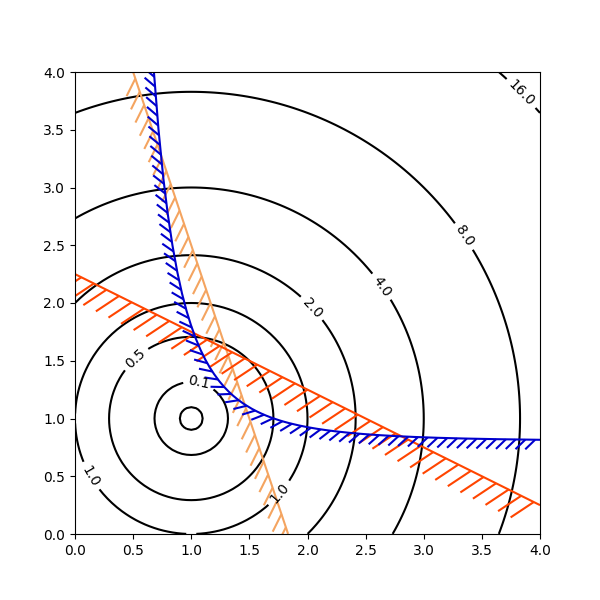# Contouring the solution space of optimizations#

Contour plotting is particularly handy when illustrating the solution space of optimization problems. Not only can axes.Axes.contour be used to represent the topography of the objective function, it can be used to generate boundary curves of the constraint functions. The constraint lines can be drawn with TickedStroke to distinguish the valid and invalid sides of the constraint boundaries.

axes.Axes.contour generates curves with larger values to the left of the contour. The angle parameter is measured zero ahead with increasing values to the left. Consequently, when using TickedStroke to illustrate a constraint in a typical optimization problem, the angle should be set between zero and 180 degrees.import numpy as np
import matplotlib.pyplot as plt
from matplotlib import patheffects

fig, ax = plt.subplots(figsize=(6, 6))

nx = 101
ny = 105

# Set up survey vectors
xvec = np.linspace(0.001, 4.0, nx)
yvec = np.linspace(0.001, 4.0, ny)

x1, x2 = np.meshgrid(xvec, yvec)

# Evaluate some stuff to plot
obj = x1**2 + x2**2 - 2*x1 - 2*x2 + 2
g1 = -(3*x1 + x2 - 5.5)
g2 = -(x1 + 2*x2 - 4.5)
g3 = 0.8 + x1**-3 - x2

cntr = ax.contour(x1, x2, obj, [0.01, 0.1, 0.5, 1, 2, 4, 8, 16],
colors='black')
ax.clabel(cntr, fmt="%2.1f", use_clabeltext=True)

cg1 = ax.contour(x1, x2, g1, , colors='sandybrown')
plt.setp(cg1.collections,
path_effects=[patheffects.withTickedStroke(angle=135)])

cg2 = ax.contour(x1, x2, g2, , colors='orangered')
plt.setp(cg2.collections,
path_effects=[patheffects.withTickedStroke(angle=60, length=2)])

cg3 = ax.contour(x1, x2, g3, , colors='mediumblue')
plt.setp(cg3.collections,
path_effects=[patheffects.withTickedStroke(spacing=7)])

ax.set_xlim(0, 4)
ax.set_ylim(0, 4)

plt.show()


Gallery generated by Sphinx-Gallery